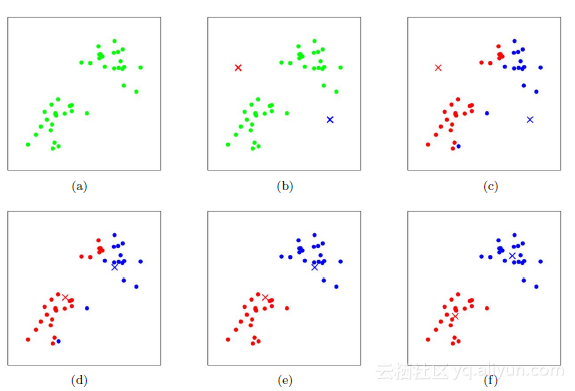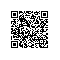# K-Means聚类算法原理

K-Means算法是无监督的聚类算法，它实现起来比较简单，聚类效果也不错，因此应用很广泛。K-Means算法有大量的变体，本文就从最传统的K-Means算法讲起，在其基础上讲述K-Means的优化变体方法。包括初始化优化K-Means++, 距离计算优化elkan K-Means算法和大数据情况下的优化Mini Batch K-Means算法。
1. K-Means原理初探
K-Means算法的思想很简单，对于给定的样本集，按照样本之间的距离大小，将样本集划分为K个簇。让簇内的点尽量紧密的连在一起，而让簇间的距离尽量的大。

$$E = \sum\limits_{i=1}^k\sum\limits_{x \in C_i} ||x-\mu_i||_2^2$$

$$\mu_i = \frac{1}{|C_i|}\sum\limits_{x \in C_i}x$$

K-Means采用的启发式方式很简单，用下面一组图就可以形象的描述。2. 传统K-Means算法流程

1）对于K-Means算法，首先要注意的是k值的选择，一般来说，我们会根据对数据的先验经验选择一个合适的k值，如果没有什么先验知识，则可以通过交叉验证选择一个合适的k值。
2）在确定了k的个数后，我们需要选择k个初始化的质心，就像上图b中的随机质心。由于我们是启发式方法，k个初始化的质心的位置选择对最后的聚类结果和运行时间都有很大的影响，因此需要选择合适的k个质心，最好这些质心不能太近。

1) 从数据集D中随机选择k个样本作为初始的k个质心向量：$\{\mu_1,\mu_2,...,\mu_k\}$
2）对于n=1,2,...,N
a) 将簇划分C初始化为$C_t = \varnothing \;\; t =1,2...k$
b) 对于i=1,2...m,计算样本$x_i$和各个质心向量$\mu_j(j=1,2,...k)$的距离：$d_{ij} = ||x_i - \mu_j||_2^2$，将$x_i$标记最小的为$d_{ij}$所对应的类别$λ_i$。此时更新$C_{\lambda_i} = C_{\lambda_i} \cup \{x_i\}$
c) 对于j=1,2,...,k,对$C_j$中所有的样本点重新计算新的质心$\mu_j = \frac{1}{|C_j|}\sum\limits_{x \in C_j}x$
e) 如果所有的k个质心向量都没有发生变化，则转到步骤3）
3） 输出簇划分$C=\{C_1,C_2,...C_k\}$
3. K-Means初始化优化K-Means++

K-Means++的对于初始化质心的优化策略也很简单，如下：
a) 从输入的数据点集合中随机选择一个点作为第一个聚类中心$μ_1$
b) 对于数据集中的每一个点$x_i$，计算它与已选择的聚类中心中最近聚类中心的距离$D(x_i) = arg\;min||x_i- \mu_r||_2^2\;\;r=1,2,...k_{selected}$
c) 选择一个新的数据点作为新的聚类中心，选择的原则是：$D(x)$较大的点，被选取作为聚类中心的概率较大
d) 重复b和c直到选择出k个聚类质心
e) 利用这k个质心来作为初始化质心去运行标准的K-Means算法
4. K-Means距离计算优化elkan K-Means

elkan K-Means利用了两边之和大于等于第三边,以及两边之差小于第三边的三角形性质，来减少距离的计算。

5. K-Means与KNN

K-Means是无监督学习的聚类算法，没有样本输出；而KNN是监督学习的分类算法，有对应的类别输出。KNN基本不需要训练，对测试集里面的点，只需要找到在训练集中最近的k个点，用这最近的k个点的类别来决定测试点的类别。而K-Means则有明显的训练过程，找到k个类别的最佳质心，从而决定样本的簇类别。使用钉钉扫一扫加入圈子
+ 订阅